# AP Chemistry : Thermodynamics

## Example Questions

### Example Question #1 : Enthalpy

Consider the following combustion reaction: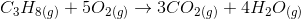The following list is the enthalpies of formation for the compounds: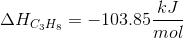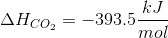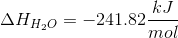If one mole of propane is burned, what is the enthalpy of the reaction?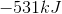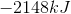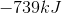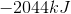Explanation:

Since we have the enthalpies of formation, we can find the enthalpy of the reaction using the following equation: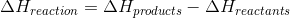We will need to use the coefficients from the balanced equation to calculate the enthalpy.Since oxygen gas is elemental, it does not have an enthalpy of formation, and is omitted from the equation.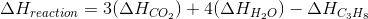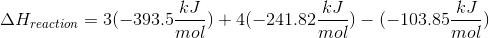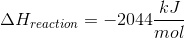### Example Question #61 : Thermochemistry And Kinetics

Which of the following is a true statement regarding the energy involved in the formation of Methane, CH4, from graphite C(s) and H2(g) ?

Energy will be released

Energy will be consumed

Energy will be consumed, then released

Energy will be released, then consumed

Energy will be consumed, then released

Explanation:

This problem requires a knowledge of the energy involved when bonds are broken versus when bonds are formed.  Energy is released when bonds are formed, and energy is consumed when bonds are broken.  Here, methane is being formed from C and H2.  Since the carbon source, graphite, is monoatomic the carbon will be involved only in the formation of C–H bonds on it's way to forming methane.  The H however exists as H2 so bonds will need to be broken to allow for C–H bonds to form.  So first energy is consumed to break the H–H bonds, it is then released when the C–H bonds form.

### Example Question #31 : Thermodynamics

H2O ice melts to liquid H2O [Heat is added]

Which of the following is correct?

I. Δ H is positive

II. ΔS is positive

III. ΔH is negative

IV. ΔS is negative

I and III

I and IV

II only

I & II

I only

I & II

Explanation:

When heat is added, enthalpy increases, ΔH refers to enthalpy

ΔS refers to entropy, which is disorder; disorder increases as it moves from solid to liquid to gas state.

As ice goes to liquid water, entropy increases

### Example Question #11 : Enthalpy

Which of the following equations is correct for finding change in enthalpy?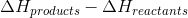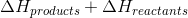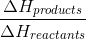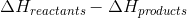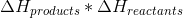Explanation:

The change in enthalpy of a reaction is determined by the amount of heat gained when the products bonds form, versus the amount of heat lost to break the bonds in the reactants. The correct formula for the enthalpy of reaction is the difference between the enthalpy of the products and that of the reactants:### Example Question #34 : Thermodynamics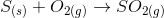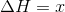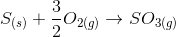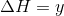Based on the given reactions, what is the enthalpy change in the following reaction?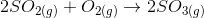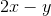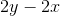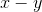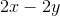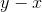Explanation:

The reactions given show the heats of formation of all the reactants and products, and we know the heat of formation of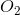is zero because it is a pure element. Formation of the product,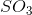gives us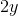. For the reactants,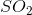gives usplusfor. Recall that the overall reaction's change in enthalpy is:Thus for this overall reaction: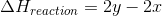### Example Question #1 : Entropy

Which of the following does not represent an increase in entropy?

Boiling water

NaCl dissolving in water

Condensation of water on glass

Sublimation of CO2

Melting of mercury

Condensation of water on glass

Explanation:

Of phases, from greatest to least entropy is gas>liquid>solid. Dissolving a salt into solution is an increase in entropy because the salt has greater space to move. The only decrease in entropy is condensation of water on glass (gas to liquid)

### Example Question #1 : Entropy

Which of the following reactions represents a negative change in entropy?

Salt dissolving

Ice melting

None of these represent a negative change in entropy

Water heating

Sublimation of dry ice

None of these represent a negative change in entropy

Explanation:

Entropy for phases are Gas>Liquid>Solid. A salt dissolving into solution has a positive entropy change (the solution becomes more chaotic). Therefore all of the listed reactions are a positive change in entropy, making E the correct answer.

### Example Question #3 : Entropy

Which of the following scenarios describes a process with a negative entropy change?

A clean room becomes cluttered

Building a skyscraper

Osmosis

An igloo melting

Building a skyscraper

Explanation:

Entropy can be thought of as the tendency for a system to become disordered, or random. Osmosis, melting ice, and a clean room becoming dirty are all examples of entropy at work. Each of these processes will occur spontaneously without the input of outside energy or work.

If you make a skyscraper, you are incorporating a series of compounds and building products into a single, distinct formation. Think of it this way: what are the odds that mother nature would spontaneously build a building? This process is very ordered, which means that entropy of the universe has decreased.

### Example Question #1 : Entropy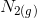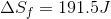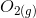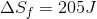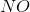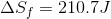What is the change in entropy for the following reaction?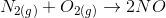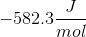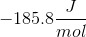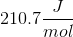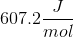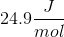Explanation:

The change in entropy is calculated by: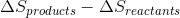When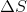cannot be measured, it can be calculated from known entropies of formation.It is important to first balance the reaction before performing calculations. The coefficients are important in determining the change in entropy of a reaction.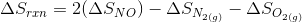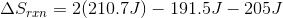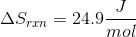### Example Question #5 : Entropy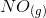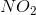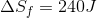What is the change in entropy for the following reaction?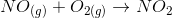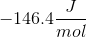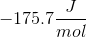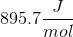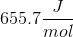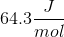Explanation:

The change in entropy is calculated by:Whencannot be measured, it can be calculated from known entropies of formation.It is important to first balance the reaction before performing calculations. The coefficients are important in determining the change in entropy of a reaction.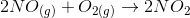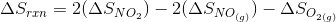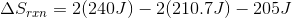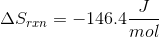### All AP Chemistry Resources